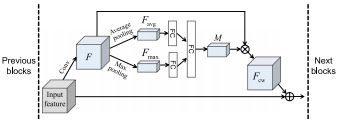# Deep network dcNet

when I tested this code

``````def forward(self,x):
out1 = self.cnn1(x)
out = self.batchnorm1(out1)
out = self.relu(out)
out = self.maxpool1(out)
out = out.view(out.size(0),-1)
out = self.fc1(out)
out2 = self.batchnorm1(out1)
out2 = self.relu(out2)
out2 = nn.AvgPool2d(out2)
out2 = self.fc2(out2)
out=torch.cat((out,out2), dim =1)

``````
``````ModuleAttributeError: 'AvgPool2d' object has no attribute 'dim'
``````

help me plzz

Hi, you initialized a layer inside your forward call.

Here is a way to deal with that:

``````import torch.nn as nn
import torch

class Model(nn.Module):
def __init__(self):
super().__init__()
self.cnn1 = nn.Conv1d(1,3, 4)
self.batchnorm1= nn.BatchNorm1d(3)
self.maxpool1 = nn.MaxPool1d(3)
self.fc1 = nn.Linear(12, 10)
self.batchnorm2 = nn.BatchNorm1d(3)
self.pool = nn.AvgPool2d(2)
self.fc2 = nn.Linear(6, 4)
self.relu = nn.ReLU()
self.flatten = nn.Flatten()
def forward(self,x):
out1 = self.cnn1(x)
out = self.batchnorm1(out1)
out = self.relu(out)
out = self.maxpool1(out)
out = self.flatten(out)
out = self.fc1(out)
out2 = self.batchnorm2(out1)
out2 = self.relu(out2)
out2 = self.pool(out2)
out2 = self.fc2(out2)
out2 = self.flatten(out2)
#print(out.shape, out2.shape)
out=torch.cat((out,out2), dim =1)
return out
m = Model()
x = torch.rand(10,1, 15)
m(x).shape == (10,14)
``````

thanks @Ouasfi but, I want to test this architecturewith this code

``````class Model(nn.Module):
def __init__(self):
super().__init__()
self.cnn1 = nn.Conv2d(in_channels=1, out_channels=8, kernel_size=3,stride=1, padding=1)
self.batchnorm1= nn.BatchNorm2d(8)
self.maxpool1 = nn.MaxPool2d(3)
self.fc1 = nn.Linear(8, 10)
self.batchnorm2 = nn.BatchNorm2d(8)
self.pool = nn.AvgPool2d(2)
self.fc2 = nn.Linear(2, 2)
self.relu = nn.ReLU()
self.flatten = nn.Flatten()
self.cnn2 = nn.Conv2d(in_channels=8, out_channels=10, kernel_size=3,stride=1, padding=1)
self.batchnorm3= nn.BatchNorm2d(10)
self.maxpool3 = nn.MaxPool2d(3)
self.fc3 = nn.Linear(8, 10)
self.batchnorm4 = nn.BatchNorm2d(8)
self.pool4 = nn.AvgPool2d(2)
self.fc4 = nn.Linear(2, 2)
self.relu4 = nn.ReLU()
self.flatten4 = nn.Flatten()
def forward(self,x):
out1 = self.cnn1(x)
out = self.batchnorm1(out1)
out = self.relu(out)
out = self.maxpool1(out)
out = self.flatten(out)
out = self.fc1(out)
out2 = self.batchnorm2(out1)
out2 = self.relu(out2)
out2 = self.pool(out2)
out2 = self.fc2(out2)
out2 = self.flatten(out2)
out=torch.cat((out,out2), dim =1)
out3 = self.cnn2(out)
out = self.batchnorm1(out3)
out = self.relu(out)
out = self.maxpool3(out)
out = self.flatten(out)
out = self.fc3(out)
out4 = self.batchnorm4(out3)
out2 = self.relu4(out4)
out2 = self.pool(out2)
out2 = self.fc2(out2)
out2 = self.flatten(out2)

#print(out.shape, out2.shape)

return out2
``````

but

``````Expected 4-dimensional input for 4-dimensional weight [10, 8, 3, 3], but got 2-dimensional input of size [6451, 42] instead
``````

helpe me plzz @Ouasfi

I corriged with this code , but it’s the same thing

``````class Model(nn.Module):
def __init__(self):
super().__init__()
self.cnn1 = nn.Conv2d(in_channels=1, out_channels=8, kernel_size=3,stride=1, padding=1)
self.batchnorm1= nn.BatchNorm2d(8)
self.maxpool1 = nn.MaxPool2d(3)
self.fc1 = nn.Linear(8, 10)
self.batchnorm2 = nn.BatchNorm2d(8)
self.pool = nn.AvgPool2d(2)
self.fc2 = nn.Linear(2, 2)
self.relu = nn.ReLU()
self.flatten = nn.Flatten()
self.cnn2 = nn.Conv2d(in_channels=8, out_channels=10, kernel_size=3,stride=1, padding=1)
self.batchnorm3= nn.BatchNorm2d(10)
self.maxpool3 = nn.MaxPool2d(3)
self.fc3 = nn.Linear(8, 10)
self.batchnorm4 = nn.BatchNorm2d(8)
self.pool4 = nn.AvgPool2d(2)
self.fc4 = nn.Linear(2, 2)
self.relu4 = nn.ReLU()
self.flatten4 = nn.Flatten()
def forward(self,x):
out1 = self.cnn1(x)
out = self.batchnorm1(out1)
out = self.relu(out)
out = self.maxpool1(out)
out = self.flatten(out)
out = self.fc1(out)
out2 = self.batchnorm2(out1)
out2 = self.relu(out2)
out2 = self.pool(out2)
out2 = self.fc2(out2)
out2 = self.flatten(out2)
out=torch.cat((out,out2), dim =1)
out3 = self.cnn2(out)
out = self.batchnorm1(out3)
out = self.relu(out)
out = self.maxpool3(out)
out = self.flatten(out)
out=torch.cat((out3,out), dim =1)
out = self.fc3(out)
out4 = self.batchnorm4(out3)
out2 = self.relu4(out4)
out2 = self.pool(out2)
out2 = self.fc2(out2)
out2 = self.flatten(out2)

#print(out.shape, out2.shape)

return out

``````

help me

help meewhat’s the input shape

the size is (7.7) @Eta_C

help meIt seems that you should reshape `out` before `self.cnn2(out)`. A convolution layer needs 4D tensor, but the output of `nn.Linear` is 2D.